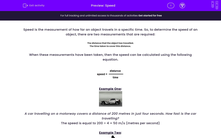# Calculate Speed

In this worksheet, students will look at how to determine the speed of an object.Key stage:  KS 3

Curriculum topic:   Physics: Motion and Forces

Curriculum subtopic:   Describing Motion

Popular topics:   Physics worksheets

Difficulty level:#### Worksheet Overview

Speed is the measurement of how far an object travels in a specific time. So, to determine the speed of an object, there are two measurements that are required:

• The distance that the object has travelled.
• The time taken to cover this distance.

When these measurements have been taken, then the speed can be calculated using the following equation.Example One:A car travelling on a motorway covers a distance of 200 metres in 4 seconds. How fast is the car travelling?

speed = distance ÷ time

Distance = 200 metres

Time = 4 seconds

So all we need to do is substitute these numbers into our equation to solve:

Speed = 200 m ÷ 4 s = 50 m/s (metres per second)

Example Two:A motorbike travels a distance of 150 miles at an average speed of 60mph. How long does this journey take?

This time, we need to rearrange the equation to make time the subject:

speed = distance ÷ time

becomes

time = distance ÷ speed

And then we just substitute our numbers into the correct places:

Distance = 150 miles

Speed = 60 mph

Time = 150 mph ÷ 60 miles = 2.5 hours

We can double-check our answer with a reverse calculation:

The speed is equal to 150 ÷ 2.5 = 60 mph (miles per hour)

Metres per second (m/s) is a commonly used unit to measure speed, but you are probably more familiar with miles per hour (mph) or kilometers per hour (kmh) when referring to the speed of cars. All of the units for speed are a distance unit divided by a time unit.

### What is EdPlace?

We're your National Curriculum aligned online education content provider helping each child succeed in English, maths and science from year 1 to GCSE. With an EdPlace account you’ll be able to track and measure progress, helping each child achieve their best. We build confidence and attainment by personalising each child’s learning at a level that suits them.

Get started